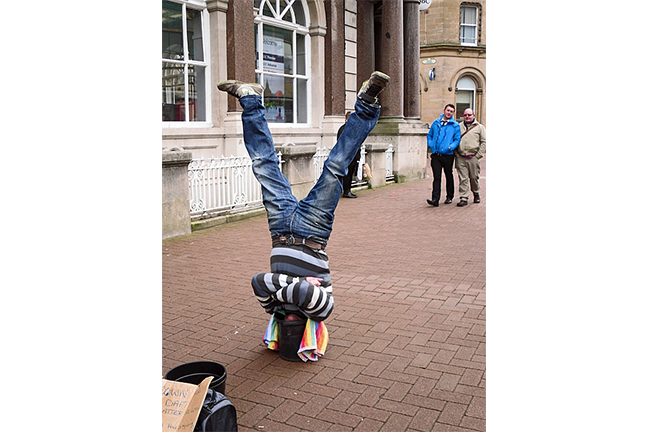## Want to keep learning?

This content is taken from the UNSW Sydney's online course, Maths for Humans: Inverse Relations and Power Laws. Join the course to learn more.
1.10

## UNSW SydneyAn inverse view

# Inverse proportionalities

An inverse relation between variable quantities $$\normalsize{x}$$ and $$\normalsize{y}$$ is given by the equation $${y=\dfrac{a}{x}}$$ for some fixed number $$\normalsize a\gt 0$$. This connects directly with the geometry of the hyperbola that we have been studying. We will see that understanding this kind of relation opens the doors to all kinds of interesting applications.

In this step, we introduce inverse relations and discuss basic examples.

## Inverse relations and symmetry

An inverse relation, or inverse proportionality is a relation of the form

$\Large{y=\frac{a}{x}}$

for some fixed constant of proportionality $$\normalsize a\gt 0$$. In this kind of situation, the bigger $$\normalsize{x}$$ gets the smaller $$\normalsize{y}$$ gets, and conversely, the smaller $$\normalsize{x}$$ gets the larger $$\normalsize{y}$$ gets. In fact by thinking about the particular inverse relation $$\normalsize{y=1/x}$$ we see that the two intervals $$\normalsize{(0,1]}$$ and $$\normalsize{[1,\infty)}$$ can be completely matched up: for every number in the first interval, its reciprocal is a number in the second interval, and vice versa. Of course this matching severely distorts measurements.

If we rewrite the basic inverse relation in the form

$\Large{xy=a}$

we see that it is completely symmetric between $$\normalsize{x}$$ and $$\normalsize{y}$$: the product of $$\normalsize{x}$$ and $$\normalsize{y}$$ is constant.

Also we see that if one of $$\normalsize{x}$$ and $$\normalsize{y}$$ is positive, so is the other one. Conversely if one is negative, then so is the other one. We can also state the relation in the form

$\Large y \propto \frac{1}{x}.$

## Speed and time

The familiar equation

$\Large{\operatorname{speed}=\frac{\operatorname{distance}}{\operatorname{time}}}$

expresses the fact that if $$\normalsize{\operatorname{distance}}$$ is constant, then $$\normalsize{\operatorname{speed}}$$ and $$\normalsize{\operatorname{time}}$$ are inversely proportional. In this discussion the terms velocity and speed are essentially synonymous.

For example suppose we want to travel $$\normalsize{10}\;\text{km}$$. If we have a speed of $$\normalsize{50\;\text{km}/\text{hr}}$$, then the time taken will be $$\normalsize{10/50=1/5}$$ hours, or $$\normalsize{12}$$ minutes. If we have a speed of $$\normalsize{100\;\text{km}/\text{hr}}$$, then the time taken will be only $$\normalsize{6}$$ minutes. If we double one quantity, the other halves. If we triple one quantity, the other is divided by three, and so on.

Q1 (E): If an Olympic walker takes $$\normalsize{3}$$ hours to walk a race, and I walk half as fast as the Olympic walker, then how long will it take me to walk the same race?

Q2 (E): If a marathon runner runs $$\normalsize{42}$$ kilometers in three hours, and you run $$\normalsize{5}$$ kilometers in $$\normalsize{45}$$ minutes, then what is the ratio between your average speed and the marathon runner’s average speed?

## Many hands make light work

Suppose we have a major construction project, involving moving lots of bricks from one place to another.Concrete blocks By Justanother GFDL or CC BY-SA 3.0, via Wikimedia Commons

Suppose it takes $$\normalsize{5}$$ people $$\normalsize{8}$$ hours to move the full load of bricks. We’ll suppose that moving bricks is an individual process, not requiring any significant cooperation. It follows that $$\normalsize{10}$$ people ought to complete the task in half the time, namely $$\normalsize{4}$$ hours. If we had $$\normalsize{20}$$ people, we could half the time again, to $$\normalsize{2}$$ hours. On the other hand, a single person would probably take about $$\normalsize{5 \times 8=40}$$ hours.

This is a good example of an inverse relation, between the number of people $$\operatorname{N}$$ to do the job, and the time $$\operatorname{T}$$ taken to do the job. And what is the specific relationship here? In this case the relationship between $$\operatorname{N}$$ and $$\operatorname{T}$$ is the inverse relation

$\Large \operatorname{T}=\frac{40}{\operatorname{N}}.$

Another way to state this is $$\operatorname{T}\times \operatorname{N}=40$$, which can be interpreted as saying that the job takes a total of 40 person-hours.

Q3 (M): If $$\normalsize{8}$$ people take $$\normalsize{5}$$ hours to paint a house, how long would it take $$\normalsize{3}$$ people to paint the same house?

The next question is a bit trickier!egg, By Hans, Free for commercial use / No attribution required

Q4 (C): If $$\normalsize{6}$$ children take $$\normalsize{5}$$ hours to paint $$\normalsize{4}$$ Easter eggs, then how long would it take $$\normalsize{5}$$ children to paint $$\normalsize{3}$$ Easter eggs?

A1. Since we halve the speed, the time doubles, and so it will take $$\normalsize{6}$$ hours to walk the same race.
A2. The average speed of the marathon runner is $$\normalsize{\frac{42\;\text{km}}{3\;\text{hr}}=14\;\text{km}/\text{hr}}$$. My average speed is $$\normalsize{\frac{5\;\text{km}}{45\;\text{min}}}$$, or equivalently, $$\normalsize{\frac{5\;\text{km}}{3/4\;\text{hr}}=20/3 \;\text{km}/\text{hr}}.$$ Therefore, the ratio of average speeds is $$\normalsize{\frac{20/3}{14}}=10/21.$$
A3. A single person will paint the house in $$\normalsize{8\times 5\;\text{hr}=40\;\text{hr}}.$$ Therefore, three people will paint the same house in $$\normalsize{40/3}\approx 13.3$$ hours.
A4. One child will paint $$\normalsize{4}$$ eggs in $$\normalsize{6\times 5\;\text{hr}=30\;\text{hr}}$$. Therefore, one child will paint one egg in $$\normalsize{30/4\;\text{hr}=15/2\;\text{hr}}$$. Thus, $$\normalsize{5}$$ children will paint one egg in $$\normalsize{\frac{15/2}{5}\;\text{hr}=3/2\;\text{hr}}$$ (this is an inverse relation), and so $$\normalsize{5}$$ children will paint $$\normalsize{3}$$ eggs in $$\normalsize{3\times 3/2\;\text{hr}=9/2\;\text{hr}}$$ (this is a direct relation).